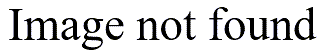# Information content of a continuous distribution

## 2005-08-01

I was browsing through the book

when I noticed that they defined the information content of the exponential distribution aswhere e is the mathematical constant 2.718… and b is the scale parameter (effectively the standard deviation) of the exponential distribution. Very interesting, I thought, since I had been working on information theory models for categorical variables and had wondered how you might extend this to continuous variables. Earlier in the book, they defined information content (or entropy) asCompare this to the formula used for categorical variablesIf you took a continuous distribution and created bins of size 1/n, the probability for bin i would beNote that with this notation, i could take on both negative and positive values, depending on the range of the distribution. For large n, this looks suspiciously like the top half of a the definition of a derivative. This tells you that the difference can be approximated bySo the entropy for a continuous variable using bins of size 1/n isThe left side of the equation is approximately equal toand the right side is the classic Riemann sum and will converge to the integral shown above. If you think about it, this is quite intuitive. You really wouldn’t want to calculate entropy for a continuous random variable the exact same way as for a categorical variable. The infinite number of values for a continuous variable would swamp the formula for entropy as derived for categorical variables. So you have to adjust for the decreasing bin widths, which is the log(n) factor seen above.

I could probably explain this better if it weren’t a Monday, so I will work on the concept a bit.

The book also computes the information content for the normal distribution. It isFor both of these distributions, a doubling of the standard deviation leads to one extra bit of uncertainty. The book does not derive the information content for a uniform distribution, but that is very easy to calculate also. If X is uniform on the interval 0 to a, then the information content of X iswhich again is very intuitive. If you cut the range of a uniform distribution in half, you have one less bit of uncertainty.